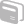Activities

•• Subject Area

• Science: Chemistry: Chemistry

• AuthorAust Senior

1 Hours

• Device
• TI-Nspire™ CAS
• Report an Issue

Moles, Molar Mass, Mass Calculations

Activity Overview

The molar masses of the most common elements have been defined in the TI-Nspire file. Using moles = mass/(molar mass) and the rearrangement of this formula, calculations are easy to perform in year 11/12 chemistry. For example, given 120g of NaCl, input 120/(Na+Cl) and the moles is calculated for you.

Before the Activity

Ensure that students have been introduced to the mole calculation concept.